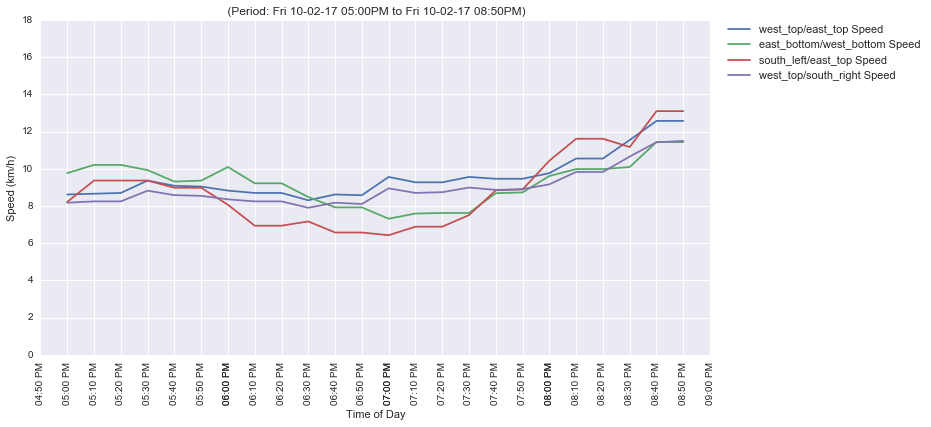In :
import matplotlib.pyplot as plt
import seaborn as sb
import mh_db as db
import mh_lib as ml
from IPython.display import display, HTML
import datetime
import pandas as pd
import numpy as np
%matplotlib inline

In :
gmaps = ml.gmaps()

In :
path_id=6
path_data = db.get_paths([path_id])
if path_data['has_segments']:
signal_segments = path_data['has_segments']
else:
signal_segments = ml.SIGNAL_SEGMENTS

signal_coords = path_data['coordinates']
segment_coords = ml.get_signal_segments_coords(path_id, signal_coords, signal_segments=signal_segments)
#for day in [8,9,10]:
#ml.run_signal_capture(gmaps, segment_coords, year=2017, month=2, day=day, start_hour=17, end_hour=21, csv='csv/signal_data.csv')

In :
HTML(data=ml.show_signal_map(path_id))

Traffic signal analysis: Big Bazaar Junction naear ITPL

Out:
In :
print("Traffic junction model")
HTML(data='<img src="https://traffic.mapshalli.org/reports/Traffic_Junction_Model.jpg"></img>')

Traffic junction model

Out:In :
(df, df_k) = ml.read_signal_csv('csv/signal_data.csv')

In :
df_k

Out:
Min Speed Mean Speed Max Speed Variation Percent
Path From/To
west_top/east_top 7.586777 9.097592 12.575342 10.411390
east_bottom/west_bottom 7.143083 9.060133 11.437975 13.326869
south_left/east_top 6.429520 8.780390 13.100752 21.265789
west_top/south_right 7.268966 8.579778 11.498182 9.277233
In :
for label in signal_segments:
df_f = df[df.name == label]
df_g = df_f.groupby(df_f.date.dt.dayofweek).mean()
ml.plot_signal_traffic(plt, df_f, by_dow=True, title=label)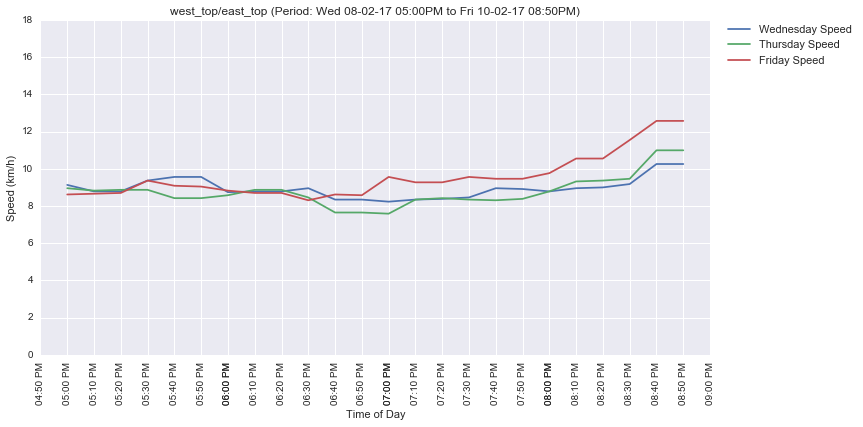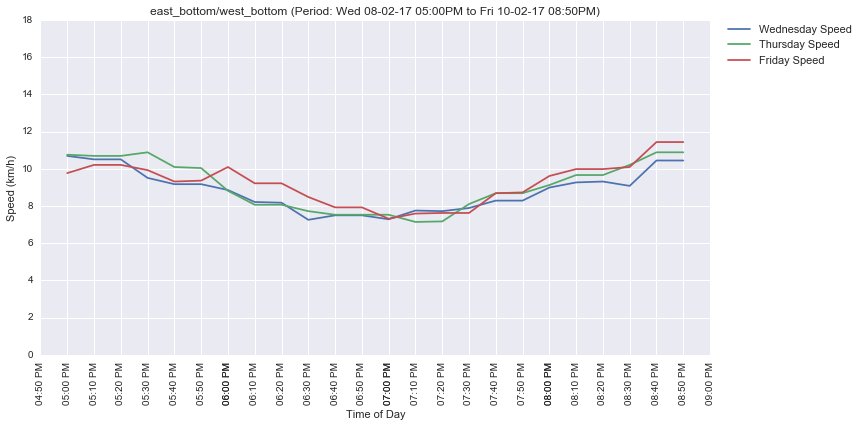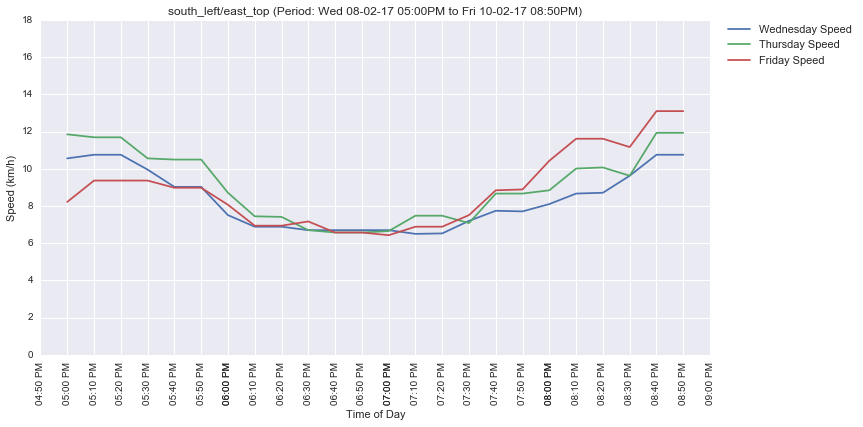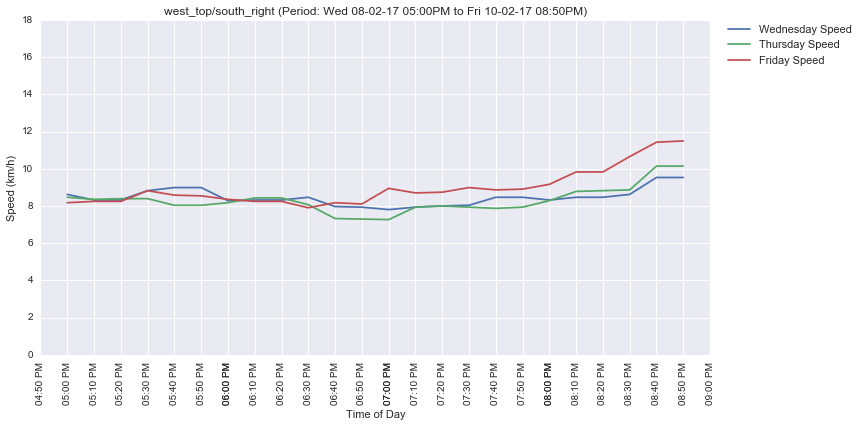In :
ml.plot_signal_traffic(plt, df[df.date.dt.dayofweek==ml.DOW['FRI']], by_labels=True, labels=signal_segments)